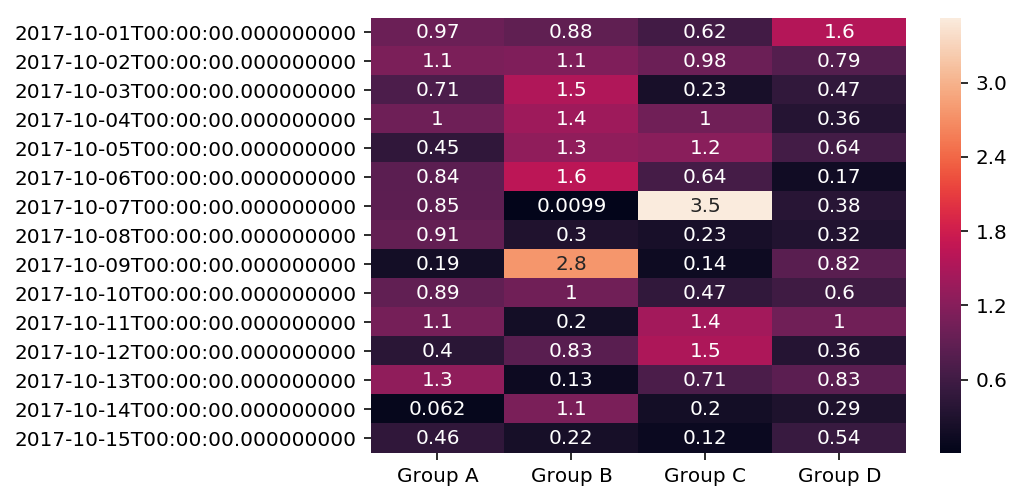01 Aug

## Heatmaps

Heatmaps are crazy useful. I use them as diagnostic plots to take a look at the feature correlation in my data frame and to understand the cross-dimensional count of my data. Basically, heatmaps tell me if there are any red flag in my data. Often really redundant data has these crazy fractal patterns in it.

### Import Preliminaries¶

In :
%matplotlib inline
%config InlineBackend.figure_format='retina'

# Import modules
import numpy as np
import pandas as pd
import seaborn as sns

# Creating the dataframe
df = pd.DataFrame()
index = pd.date_range(start='2017-10-1', end='2017-10-15', freq='D')
cols = ['Group A', 'Group B','Group C','Group D']
df = pd.DataFrame(data = abs(np.random.randn(15,4)),
index=index, columns=cols )
# View the dataframe

Out:
Group A Group B Group C Group D
2017-10-01 0.972536 0.877120 0.621920 1.567712
2017-10-02 1.113678 1.138295 0.976972 0.789277
2017-10-03 0.714975 1.543649 0.231906 0.470471
2017-10-04 0.998933 1.391118 1.019826 0.359245
2017-10-05 0.454609 1.274193 1.218750 0.636881

### Heatmap¶

In :
# plot a heatmap using seaborn
sns.heatmap(df,annot=True);Author: Karvi Sekhon